Courses

# Electrochemistry (Chapter Test - Medical)

## 20 Questions MCQ Test | Electrochemistry (Chapter Test - Medical)

Description
This mock test of Electrochemistry (Chapter Test - Medical) for Class 12 helps you for every Class 12 entrance exam. This contains 20 Multiple Choice Questions for Class 12 Electrochemistry (Chapter Test - Medical) (mcq) to study with solutions a complete question bank. The solved questions answers in this Electrochemistry (Chapter Test - Medical) quiz give you a good mix of easy questions and tough questions. Class 12 students definitely take this Electrochemistry (Chapter Test - Medical) exercise for a better result in the exam. You can find other Electrochemistry (Chapter Test - Medical) extra questions, long questions & short questions for Class 12 on EduRev as well by searching above.
QUESTION: 1

Solution:
QUESTION: 2

Solution:
QUESTION: 3

### Acidified water is electrolysed by using inert electrodes. The volume of gases liberated at STP is 168 mL. The quantity of charge passed is

Solution:
QUESTION: 4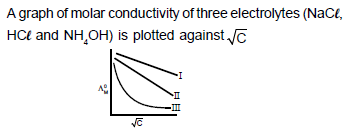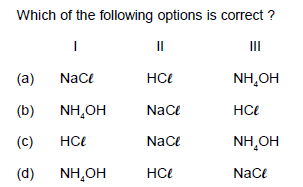Solution:
QUESTION: 5

Which of the following statement is incorrect about electrolysis of AgNO3 using silver electrodes ?

Solution:
QUESTION: 6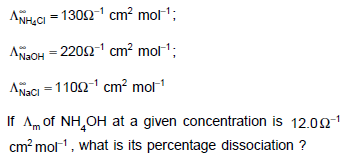Solution:
QUESTION: 7

A decinormal solution of NaCl has specific conductance k equal to 9.2 x 10-3 S cm-1. If ionic conductance of Na+ and Cl- at the same temperature are 43 and 65 S cm-2 mol-1 respectively, the degree of dissociation is

Solution:
QUESTION: 8

Q. The molar conductivities of NaOH, NaCl and BaCl2 at infinite dilution are 2⋅481×10−2Sm2mol−1,1⋅265×10−2Sm2mol−1 and 2⋅800×10−2Sm2mol−1 respectively. The molar conductivity of Ba(OH)2 at infinite dilution will be

Solution:

[Ba(OH)2]
= ⋀m(BaCl2) + 2 ⋀m(NaOH) − 2⋀m(NaCl)

= (2⋅8 + 2 × 2⋅481 − 2 × 1⋅265) × 10−2Sm2mo−11
= (2⋅8 + 4⋅962 − 2⋅53 )× 10−2Sm2mol−1

= 5⋅232×10−2Sm2mol−1

QUESTION: 9

Molar conductivity of a solution is 1.26 x 102 ohm-1 cm2 mol-1. Its molarity is 0.01, its specific conductivity will be

Solution:
QUESTION: 10

If the solution of the CuSO4 in which copper rod is immersed is diluted to 10 times, the electrode potential

Solution:
QUESTION: 11

2A- + B2 → 2B+ A2 :

2C- + B→ No reaction

2D+ A2 → 2A- + D2,

Which of the following is correct?

Solution:
QUESTION: 12

Which of the following is the electrolysis reaction when AgNO3(aq) is electrolysed using a silver anode and a
platinum cathode ?

Solution:
QUESTION: 13

Which Nernst equation is true to find out the potential of non-standard electrochemical cell from the following?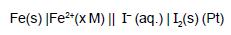Solution:
QUESTION: 14

The molar conductances of NaCI, HCI and CH3COONa at infinite dilution are 126.45, 426.16 and 91 ohm-1 cm2 mol–1 respectively. The molar conductance of CH3COOH at infinite dilution is

Solution:
QUESTION: 15

E1 , E2 and E3 are the emf values of the three galvanic cells respectively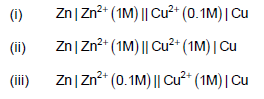Which one of the following is true ?

Solution:
QUESTION: 16

If the half-cell reaction, A + e- ® A- has a large
negative reduction potential, it follows that

Solution:
QUESTION: 17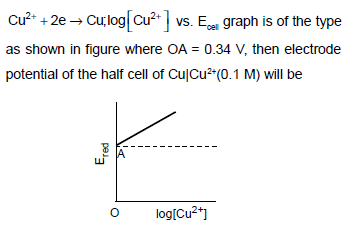Solution:
QUESTION: 18

A standard hydrogen electrode has zero electrode potential because

Solution:
QUESTION: 19

When electric current is passed through a cell having an electrolyte, the positive ions move towards cathode
and the negative ions towards the anode. If the cathode is removed from the solution then

Solution:
QUESTION: 20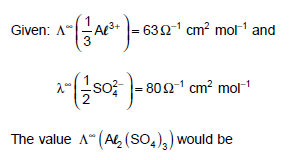Solution: